# Converting nested JSON structures to Pandas DataFrames

• Last Updated : 22 Nov, 2021

In this article, we are going to see how to convert nested JSON structures to Pandas DataFrames.

## JSON with multiple levels

In this case, the nested JSON data contains another JSON object as the value for some of its attributes. This makes the data multi-level and we need to flatten it as per the project requirements for better readability, as explained below.

## Python3

 `# importing the libraries used``import` `pandas as pd`` ` `# initializing the data``data ``=` `{``    ``'company'``: ``'XYZ pvt ltd'``,``    ``'location'``: ``'London'``,``    ``'info'``: {``        ``'president'``: ``'Rakesh Kapoor'``,``        ``'contacts'``: {``            ``'email'``: ``'contact@xyz.com'``,``            ``'tel'``: ``'9876543210'``        ``}``    ``}``}`

Here, the data contains multiple levels. To convert it to a dataframe we will use the json_normalize() function of the pandas library.

## Python3

 `pd.json_normalize(data)`

Output:json data converted to pandas dataframe

Here, we see that the data is flattened and converted to columns. If we do not wish to completely flatten the data, we can use the max_level attribute as shown below.

## Python3

 `pd.json_normalize(data,max_level``=``0``)`

Output: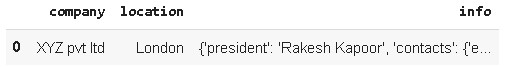json data converted to pandas dataframe

Here, we see that the info column is not flattened further.

## Python3

 `pd.json_normalize(data,max_level``=``1``)`

Output: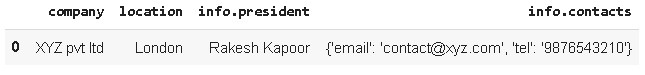json data converted to pandas dataframe

Here, we see that the contacts column is not flattened further.

## List of nested JSON

Now, if the data is a list of nested JSONs, we will get multiple records in our dataframe.

## Python3

 `data ``=` `[``    ``{``        ``'id'``: ``'001'``,``        ``'company'``: ``'XYZ pvt ltd'``,``        ``'location'``: ``'London'``,``        ``'info'``: {``            ``'president'``: ``'Rakesh Kapoor'``,``            ``'contacts'``: {``                    ``'email'``: ``'contact@xyz.com'``,``                    ``'tel'``: ``'9876543210'``            ``}``        ``}``    ``},``    ``{``        ``'id'``: ``'002'``,``        ``'company'``: ``'PQR Associates'``,``        ``'location'``: ``'Abu Dhabi'``,``        ``'info'``: {``            ``'president'``: ``'Neelam Subramaniyam'``,``            ``'contacts'``: {``                    ``'email'``: ``'contact@pqr.com'``,``                    ``'tel'``: ``'8876443210'``            ``}``        ``}``    ``}``]`` ` `pd.json_normalize(data)`

Output: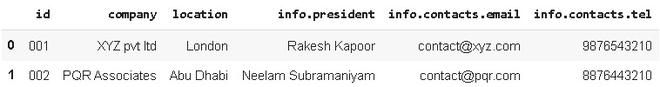json data converted to pandas dataframe

So, in the case of multiple levels of JSON, we can try out different values of max_level attribute.

## JSON with nested lists

In this case, the nested JSON has a list of JSON objects as the value for some of its attributes. In such a case, we can choose the inner list items to be the records/rows of our dataframe using the record_path attribute.

## Python3

 `# initialising the data``data ``=` `{``    ``'company'``: ``'XYZ pvt ltd'``,``    ``'location'``: ``'London'``,``    ``'info'``: {``        ``'president'``: ``'Rakesh Kapoor'``,``        ``'contacts'``: {``            ``'email'``: ``'contact@xyz.com'``,``            ``'tel'``: ``'9876543210'``        ``}``    ``},``    ``'employees'``: [``        ``{``'name'``: ``'A'``},``        ``{``'name'``: ``'B'``},``        ``{``'name'``: ``'C'``}``    ``]``}`` ` `# converting the data to dataframe``df ``=` `pd.json_normalize(data)`

Output: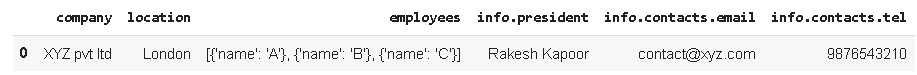json data converted to pandas dataframe

Here, the nested list is not flattened. We need to use record_path  attribute to flatten the nested list.

## Python3

 `pd.json_normalize(data,record_path``=``[``'employees'``])`

Output: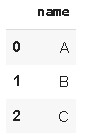nested list is not flattened

Now, we observe that it does not include ‘info’ and other features. To include them we use another attribute, meta.  Note that, in the below code, to include an attribute of an inner JSON we have specified the path as  “[‘info’, ‘president’]”.

## Python3

 `pd.json_normalize(data, record_path``=``[``'employees'``], meta``=``[``                  ``'company'``, ``'location'``, [``'info'``, ``'president'``]])`

Output: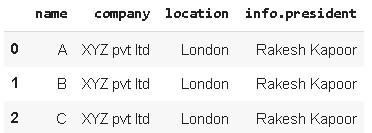json data converted to pandas dataframe

Now in the case of multiple nested JSON objects, we will get a dataframe with multiple records as shown below.

## Python3

 `data ``=` `[``    ``{``        ``'id'``: ``'001'``,``        ``'company'``: ``'XYZ pvt ltd'``,``        ``'location'``: ``'London'``,``        ``'info'``: {``            ``'president'``: ``'Rakesh Kapoor'``,``            ``'contacts'``: {``                    ``'email'``: ``'contact@xyz.com'``,``                    ``'tel'``: ``'9876543210'``            ``}``        ``},``        ``'employees'``: [``            ``{``'name'``: ``'A'``},``            ``{``'name'``: ``'B'``},``            ``{``'name'``: ``'C'``}``        ``]``    ``},``    ``{``        ``'id'``: ``'002'``,``        ``'company'``: ``'PQR Associates'``,``        ``'location'``: ``'Abu Dhabi'``,``        ``'info'``: {``            ``'president'``: ``'Neelam Subramaniyam'``,``            ``'contacts'``: {``                    ``'email'``: ``'contact@pqr.com'``,``                    ``'tel'``: ``'8876443210'``            ``}``        ``},``        ``'employees'``: [``            ``{``'name'``: ``'L'``},``            ``{``'name'``: ``'M'``},``            ``{``'name'``: ``'N'``}``        ``]``    ``}``]`` ` `df ``=` `pd.json_normalize(data, record_path``=``[``'employees'``], meta``=``[``                       ``'company'``, ``'location'``, [``'info'``, ``'president'``]])``print``(df)`

Output :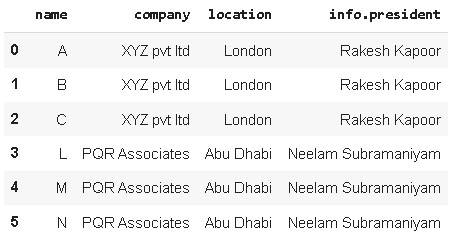json data converted to pandas dataframe

My Personal Notes arrow_drop_up